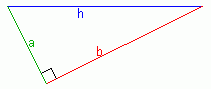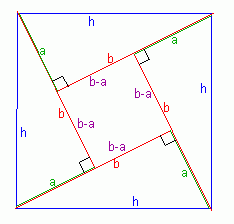SEARCH HOMEMath Central Quandaries & QueriesQuestion from VINEET, a student: in a right angle triangle, hypotenous side is less than the sum of other two sides, how the square of hypotenous is equal to the sum of squares of other tqo sides?Hi Vineet.

I'm not certain whether you are wondering:

1) Why does the Pythagorean Theorem work? or

2) Why does squaring the numbers suddenly made things go from a less than to an equal to?

So I'll try to answer both questions.

Why does the Pythagorean Theorem work?

Let's say you have a right-angled triangle whose hypotenuse length is h and whose other sides are a and b, where a≤b (a is shorter or the same length as b). This can describe any right angled triangle.Now let's draw four of them with a square in the middle as follows:Now you can see I've created a square with h on each side. This means the area of the whole big diagram is h2 . But you have the same area as if you add up the areas of the central small square and the four triangular areas. The area of a triangle is half the base times the height, so the area of one triangle is ½ab. The central square has the area of (b-a)2 . This means that:

h2 = 4( ½ab ) + (b-a)2

so let's solve this:

h2 = 2ab + (b2 - 2ab + a2)

h2 = a2 + b2

And this is the Pythagorean Theorem we've just proven!

Why does squaring the numbers suddenly made things go from a less than to an equal to?

Let's look at the two equations we have:

h < a + b and

h2 = a2 + b2

Remember that these are all length measurements, so they are all positive.

What happens if we square both sides of the inequality? We get:

h2 < (a + b)2

that's

h2 < a2 + 2ab + b2

but if h2 = a2 + b2 for a right triangle, then we can substitute this for h2 in the inequality:

a2 + b2 < a2 + 2ab + b2

simplify it and you get

0 < 2ab

which is certainly true since a and b are both positive numbers.

So this is showing how squaring the sum of two positive numbers is larger than the sum of the squares of the same two numbers. That means that even though the unsquared condition might be a less-than relationship, the squared condition can be equal-to in a right triangle.

Hope this helps,
Stephen La Rocque.Math Central is supported by the University of Regina and The Pacific Institute for the Mathematical Sciences.